## Excel VARA function

The VARA function calculates the variance based on a given sample.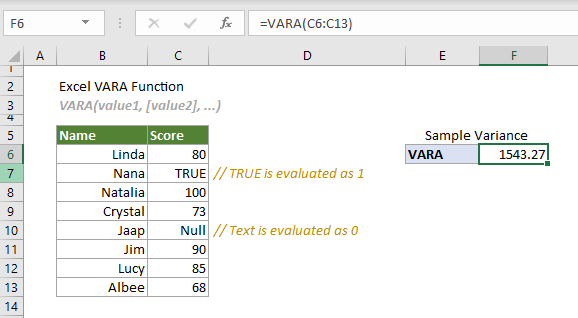#### VARA function vs. VAR.S function

Both the VARA function and VAR.S function can be used to estimate the variance based on given sample. The difference between them is that the VARA function evaluates text values and logical values in references, whereas VAR.S function does not include text values and logical values in references as a part of the calculation.

#### Excel variance functions

The following table lists all Excel variance functions and their usage notes, which can help you choose the one that best suits your needs.

 Name Data type Text and logicals in references VAR Sample Ignored VAR.S Sample Ignored VARA Sample Evaluated VARP Population Ignore VAR.P Population Ignored VARPA Population Evaluated

#### Syntax

VAR(value1, [value2], ...)

#### Arguments

• Value1 (required): The first sample value corresponding to the population.
• Value2, ... (required): The second and more sample values corresponding to the population.

#### Remarks

1. There can be up to 255 arguments at a time.
2. Arguments can be either of:
-- Numbers;
-- Range names, arrays or cell references that contain numbers.
3. The VARA function takes the arguments as a sample of the population.
Tips: If the data you provided represents the whole population, it is recommended to use the VARPA function.
4. Logical values and text representations of numbers that you directly type in the arguments will be included in the calculation:
-- Logical values: TRUE (1) or FALSE (0);
-- Text representations of numbers: Numbers enclosed in double quotation marks such as “2”.
5. By default, VARA function evaluates logical values and text values in references.
-- Logical values: TRUE is evaluated as 1, and FALSE is evaluated as 0.
-- Text value: is evaluated as 0.
Tips: If you don’t want to include logical values and text values in references as part of the calculation, it is recommended to use the VAR.S function.
6. Empty cells, logical values, text, or error values will be ignored in the array or cell references.
7. The #VALUE! error occurs if any of the supplied arguments you typed directly in the function are text strings that cannot be interpreted as numbers by Excel.
8. The #DIV/0! error occurs if there are less than 2 numeric values supplied to the function.
9. The equation of the VARA function is as follows: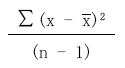Where
-- x represents each value in the sample.
--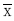is the average of the sample.
-- n is the number of cells in the sample range.

#### Return value

It returns a numeric value.

#### Example

The following table is a sample of scores. To estimate the variance based on it, while needing to evaluate the logical value and text value in the referenced cells, you can apply the VARA function to handle it.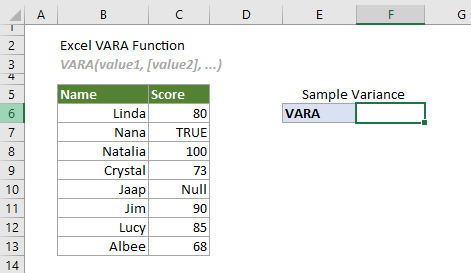Select a cell (says F6 in this case), enter the formula below and press the Enter key to get the variance.

=VARA(C6:C13)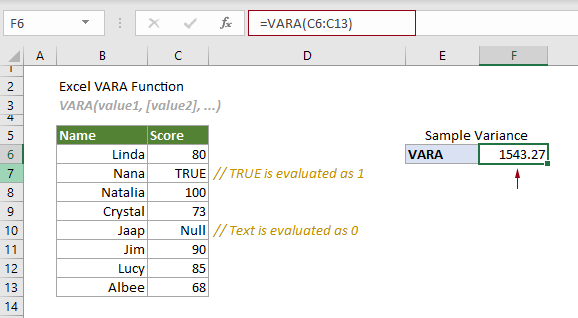Notes:

1. The argument in the formula above is supplied as a cell range.

1) The logical value “TRUE” in the reference is evaluated as 1.
2) The text “Null” in the reference is evaluated as 0.
3) The formula can be changed to:
=VARA(C6,C7,C8,C9,C10,C11,C12,C13)
=VARA(C6,1,C8,C9,0,C11,C12,C13)

2. In this case, if you apply the VAR.S function to estimate the variance based on a given sample in C6:C13, the logical value and the text in the reference will be ignored. See the following screenshot: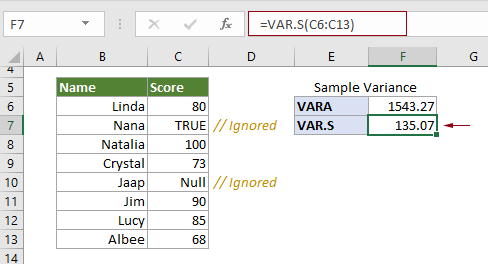#### Related Functions

Excel VAR.P function
The VAR.P function calculates the variance based on the entire population.

Excel VAR function
The VAR function calculates the variance based on a given sample.

Excel VAR.S function
The VAR.S function calculates the variance based on a given sample.

Excel VARP function
The VARP function calculates the variance based on the entire population.

Excel VARPA function
The VARPA function calculates the variance based on the entire population.

### The Best Office Productivity Tools

#### Kutools for Excel - Helps You To Stand Out From Crowd

Would you like to complete your daily work quickly and perfectly? Kutools for Excel brings 300 powerful advanced features (Combine workbooks, sum by color, split cell contents, convert date, and so on...) and save 80% time for you.

• Designed for 1500 work scenarios, helps you solve 80% Excel problems.
• Reduce thousands of keyboard and mouse clicks every day, relieve your tired eyes and hands.
• Become an Excel expert in 3 minutes. No longer need to remember any painful formulas and VBA codes.
• 30-day unlimited free trial. 60-day money back guarantee. Free upgrade and support for 2 years.#### Office Tab - Enable Tabbed Reading and Editing in Microsoft Office (include Excel)

• One second to switch between dozens of open documents!
• Reduce hundreds of mouse clicks for you every day, say goodbye to mouse hand.
• Increases your productivity by 50% when viewing and editing multiple documents.
• Brings Efficient Tabs to Office (include Excel), Just Like Chrome, Firefox, And New Internet Explorer.